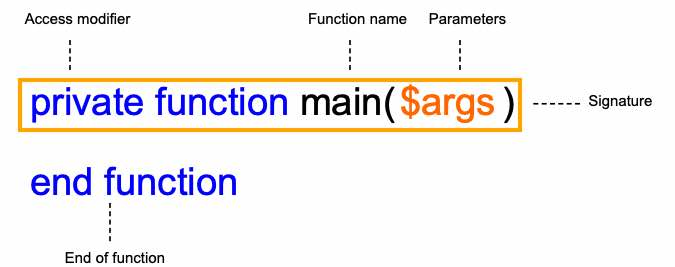Jamie Balfour

Welcome to my personal website.

Find out more about me, my personal projects, reviews, courses and much more here.

# Part 3.2Functions in YASS

The concept of sub-programming, i.e. programs or chunks of code within another larger program was developed in the aim to make a program more readable. By introducing this concept into the many programming languages out there, programs became more modular.

## What a function is

A function in programming is something that does something else when given some input.

A function may return (output) something when it is finished processing this input. A function can be given parameters so that it can receive arguments (values to the parameters). When a function is needed it is called.

A function is similar to a method, except that normally methods are applied to objects.

## YASS functions

A YASS function is a syntax that encapsulates code within it. As mentioned, like many languages, YASS may choose to return something when it completes its execution.

Every YASS program starts with a main function - even those that do not include them are compiled to include one. The main function is always the first part of code to be run by ZPE.

A function looks like:The syntax of a function in YASS

The access modifier is used to determine what other functions can access the function, they are private, public and default. Default is achieved by not specifying an access modifier at all.

The following is an example of a main function:

YASS
function main($args) print("Hello world!") end function  The following method contains two functions, a main function and a hello function: YASS function hello() print("Hello world!") end function function main($args)
//Call the hello function
hello()
end function


In this example the main function calls the hello function.

### Passing parameters in YASS

Functions may also have parameters passed to them. These are known as arguments or formal parameters when they are received.

The next example includes two functions, a main function and an add function but this time the function also has parameters passed to it:

YASS
function add($num1,$num2)
print($num1 +$num2)
end function

function main($args) //Call the add function add(10, 20) end function  In this example the main function calls the add function. ### Returning a value Returning a value is in essence a function giving something back to whatever part of the program originally called the function. This allows functions to act a processors for a small chunk of code then provide an output that can be further processed. In the following sample, the print function call has been replaced with a return to give back the result and the main function stores the result in a variable called $result before printing that variable:

YASS
function add($num1,$num2)
return $num1 +$num2
end function

function main($args) //Call the add function$result = add(10, 20)
print($result) end function  ## Anonymous functions Anonymous functions or lambda functions are a method of declaring a function without a name. These functions themselves can be assigned to a varaible or object property, but they do not have names. These kinds of functions are seen across a variety of languages ranging from JavaScript to Python and PHP and have become very popular since their introduction. YASS uses these functions for a huge range of in-built functions. An anonymous function is defined as a function without a name, so to create one all that differs from an ordinary function is that there is no name following the keyword function: YASS function ($x, $y) return 5 *$x * $y end function  These functions can be assigned to variables and then called: YASS $f = function ($x,$y)
return 5 * $x *$y
end function

print($f(5, 2))  Anonymous functions can also be embedded as arguments for other functions. For example with the YASS built-in function csv_decode function: YASS $out = csv_decode($content, function($x) return $x + 5 end function) print($out)

Feedback 👍
Comments are sent via email to me.